## Partial Differential Equation

A partial differential equation (PDE) is an equation involving functions and their Partial Derivatives; for example, the Wave Equation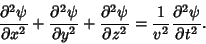(1)

In general, partial differential equations are much more difficult to solve analytically than are Ordinary Differential Equations. They may sometimes be solved using a Bäcklund Transformation, Characteristic, Green's Function, Integral Transform, Lax Pair, Separation of Variables, or--when all else fails (which it frequently does)--numerical methods.

Fortunately, partial differential equations of second-order are often amenable to analytical solution. Such PDEs are of the form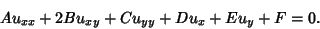(2)

Second-order PDEs are then classified according to the properties of the Matrix(3)

as Elliptic, Hyperbolic, or Parabolic.

If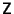is a Positive Definite Matrix, i.e., det, the PDE is said to be Elliptic. Laplace's Equation and Poisson's Equation are examples. Boundary conditions are used to give the constrainton, where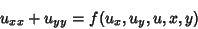(4)

holds in.

If det, the PDE is said to be Hyperbolic. The Wave Equation is an example of a hyperbolic partial differential equation. Initial-boundary conditions are used to give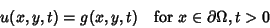(5)(6)(7)

where(8)

holds in.

If det, the PDE is said to be parabolic. The Heat Conduction Equation equation and other diffusion equations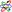are examples. Initial-boundary conditions are used to give(9)(10)

where(11)

holds in.

See also Bäcklund Transformation, Boundary Conditions, Characteristic (Partial Differential Equation), Elliptic Partial Differential Equation, Green's Function, Hyperbolic Partial Differential Equation, Integral Transform, Johnson's Equation, Lax Pair, Monge-Ampère Differential Equation, Parabolic Partial Differential Equation, Separation of Variables

References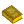Partial Differential Equations

Arfken, G. Partial Differential Equations of Theoretical Physics.'' §8.1 in Mathematical Methods for Physicists, 3rd ed. Orlando, FL: Academic Press, pp. 437-440, 1985.

Bateman, H. Partial Differential Equations of Mathematical Physics. New York: Dover, 1944.

Press, W. H.; Flannery, B. P.; Teukolsky, S. A.; and Vetterling, W. T. Partial Differential Equations.'' Ch. 19 in Numerical Recipes in FORTRAN: The Art of Scientific Computing, 2nd ed. Cambridge, England: Cambridge University Press, pp. 818-880, 1992.

Sobolev, S. L. Partial Differential Equations of Mathematical Physics. New York: Dover, 1989.

Sommerfeld, A. Partial Differential Equations in Physics. New York: Academic Press, 1964.

Webster, A. G. Partial Differential Equations of Mathematical Physics, 2nd corr. ed. New York: Dover, 1955.